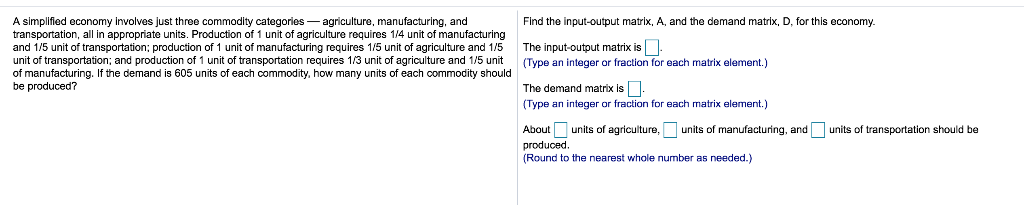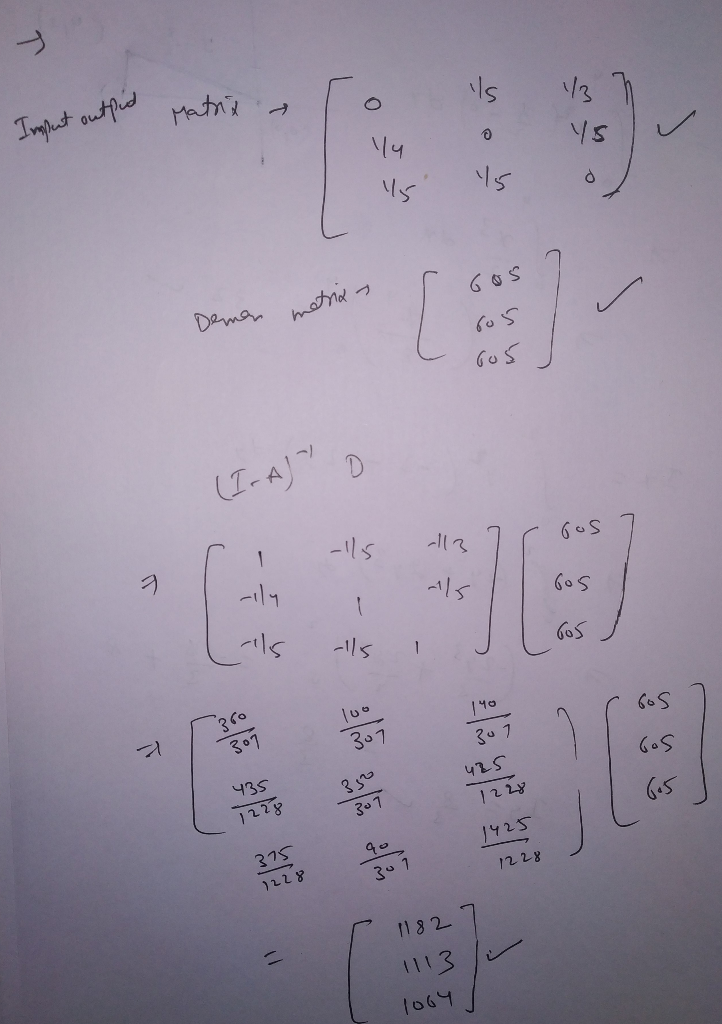Homework Help Question & Answers

1 Find the input-output matrix, A, and the demand matrix, D, for this economy. A simplified econo...1 Find the input-output matrix, A, and the demand matrix, D, for this economy. A simplified economy involves just three commodity categories agriculture, manufacturing, and transportation, all in appropriate units. Production of 1 unit of agriculture requires 14 unit of manufacturing and 1/5 unit of transportation; production of 1 unit of manufacturing requires 1/5 unit of agriculture and 1/5 The input-output matrix is unit of transportation; and production of 1 unit of transportation requires 13 unit of agriculture and 1/5 unit (Type an integer or fraction for each matrix element.) of manufacturing. If the demand is 605 units of each commodity, how many units of each commodity should be produced? The demand matrix is (Type an integer or fraction for each matrix element.) Aboutunits of agriculture,units of manufacturing, and produced. Round to the nearest whole number as needed.) units of transportation should be

Any problem in any answer then comment bellow...So 1182 units of agriculture , 1114 units of manufacturing and 1064 unitsbof transportation...

Add Answer of: 1 Find the input-output matrix, A, and the demand matrix, D, for this economy. A simplified econo...
More Homework Help Questions Additional questions in this topic.

• Derived demand refers to a) demand curves derived from utility functions b) an individual demand curve estimated from a market demand curve c) a market demand curve estimated from individual demand curves d) demand for a resource derived from the demand

Need Online Homework Help?

Get FREE EXPERT Answers
WITHIN MINUTES
Related Questions